# Geodesic Bending Invariants for Surfaces¶

$\newcommand{\dotp}{\langle #1, #2 \rangle}$ $\newcommand{\enscond}{\lbrace #1, #2 \rbrace}$ $\newcommand{\pd}{ \frac{ \partial #1}{\partial #2} }$ $\newcommand{\umin}{\underset{#1}{\min}\;}$ $\newcommand{\umax}{\underset{#1}{\max}\;}$ $\newcommand{\umin}{\underset{#1}{\min}\;}$ $\newcommand{\uargmin}{\underset{#1}{argmin}\;}$ $\newcommand{\norm}{\|#1\|}$ $\newcommand{\abs}{\left|#1\right|}$ $\newcommand{\choice}{ \left\{ \begin{array}{l} #1 \end{array} \right. }$ $\newcommand{\pa}{\left(#1\right)}$ $\newcommand{\diag}{{diag}\left( #1 \right)}$ $\newcommand{\qandq}{\quad\text{and}\quad}$ $\newcommand{\qwhereq}{\quad\text{where}\quad}$ $\newcommand{\qifq}{ \quad \text{if} \quad }$ $\newcommand{\qarrq}{ \quad \Longrightarrow \quad }$ $\newcommand{\ZZ}{\mathbb{Z}}$ $\newcommand{\CC}{\mathbb{C}}$ $\newcommand{\RR}{\mathbb{R}}$ $\newcommand{\EE}{\mathbb{E}}$ $\newcommand{\Zz}{\mathcal{Z}}$ $\newcommand{\Ww}{\mathcal{W}}$ $\newcommand{\Vv}{\mathcal{V}}$ $\newcommand{\Nn}{\mathcal{N}}$ $\newcommand{\NN}{\mathcal{N}}$ $\newcommand{\Hh}{\mathcal{H}}$ $\newcommand{\Bb}{\mathcal{B}}$ $\newcommand{\Ee}{\mathcal{E}}$ $\newcommand{\Cc}{\mathcal{C}}$ $\newcommand{\Gg}{\mathcal{G}}$ $\newcommand{\Ss}{\mathcal{S}}$ $\newcommand{\Pp}{\mathcal{P}}$ $\newcommand{\Ff}{\mathcal{F}}$ $\newcommand{\Xx}{\mathcal{X}}$ $\newcommand{\Mm}{\mathcal{M}}$ $\newcommand{\Ii}{\mathcal{I}}$ $\newcommand{\Dd}{\mathcal{D}}$ $\newcommand{\Ll}{\mathcal{L}}$ $\newcommand{\Tt}{\mathcal{T}}$ $\newcommand{\si}{\sigma}$ $\newcommand{\al}{\alpha}$ $\newcommand{\la}{\lambda}$ $\newcommand{\ga}{\gamma}$ $\newcommand{\Ga}{\Gamma}$ $\newcommand{\La}{\Lambda}$ $\newcommand{\si}{\sigma}$ $\newcommand{\Si}{\Sigma}$ $\newcommand{\be}{\beta}$ $\newcommand{\de}{\delta}$ $\newcommand{\De}{\Delta}$ $\newcommand{\phi}{\varphi}$ $\newcommand{\th}{\theta}$ $\newcommand{\om}{\omega}$ $\newcommand{\Om}{\Omega}$

This tour explores the computation of bending invariants of surfaces.

In :
addpath('toolbox_signal')


## Bending Invariants¶

Bending invariants replace the position of the vertices in a shape $\Ss$ (2-D or 3-D) by new positions that are insensitive to isometric deformation of the shape. This defines a bending invariant signature that can be used for surface matching.

Bending invariant were introduced in EladKim03. A related method was developped for brain flattening in SchwShWolf89. This method is related to the Isomap algorithm for manifold learning TenSolvLang03.

We assume that $Ss$ has some Riemannian metric, for instance coming from the embedding of a surface in 3-D Euclidian space, or by restriction of the Euclian 2-D space to a 2-D sub-domain (planar shape). One thus can compute the geodesic distance $d(x,x')$ between points $x,x' \in \Ss$.

The bending invariant $\tilde \Ss$ of $\Ss$ is defined as the set of points $Y = (y_i)_j \subset \RR^d$ that are optimized so that the Euclidean distance between points in $Y$ matches as closely the geodesic distance between points in $X$, i.e. $$\forall i, j, \quad \norm{y_i-y_j} \approx d(x_i,x_j)$$

Multi-dimensional scaling (MDS) is a class of method that aims at computing such a set of points $Y \in \RR^{d \times N}$ in $\RR^d$ such that $$\forall i, j, \quad \norm{y_i-y_j} \approx \de_{i,j}$$ where $\de \in \RR^{N \times N}$ is a input data matrix. For a detailed overview of MDS, we refer to the book BorgGroe97

In this tour, we apply two specific MDS algorithms (strain and stress minimization) with input $\de_{i,j} = d(x_i,x_j)$.

## 3-D Surfaces and Geodesic Distances¶

We consider here a syrface $\Ss \subset \RR^3$.

Load a mesh of $N$ vertices that discretizes this surfaces.

In :
name = 'camel';
options.name = name;
N = size(V,2);


Display it.

In :
clf;
plot_mesh(V,F, options);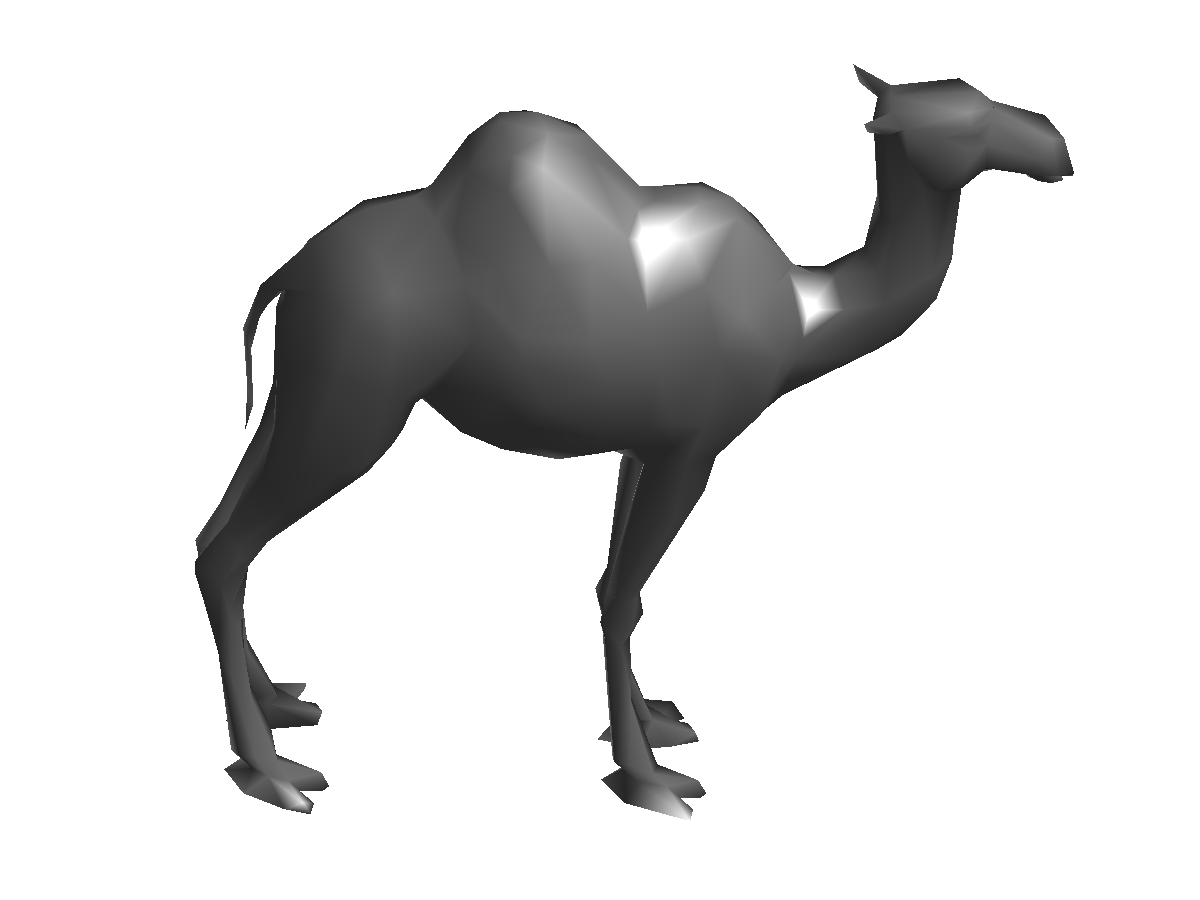The geodesic distance map $U(x) = d(x,x_i)$ to a starting point $x_i$ can be computed in $O(N \log(N))$ operations on a mesh of $N$ vertices using the Fast Marching algorithm.

In :
i = 1;
U = perform_fast_marching_mesh(V, F, i);


Extract a bunch of geodesic shortest paths from $x_i$ to randomly selected vertices $(x_j)_{j \in J}$.

In :
options.method = 'continuous';
J = randperm(N); J = J(1:50);
paths = compute_geodesic_mesh(U, V, F, J, options);


Display the distance $U$ on the 3-D mesh together with the geodesic paths.

In :
clf;
plot_fast_marching_mesh(V, F, U, paths, options);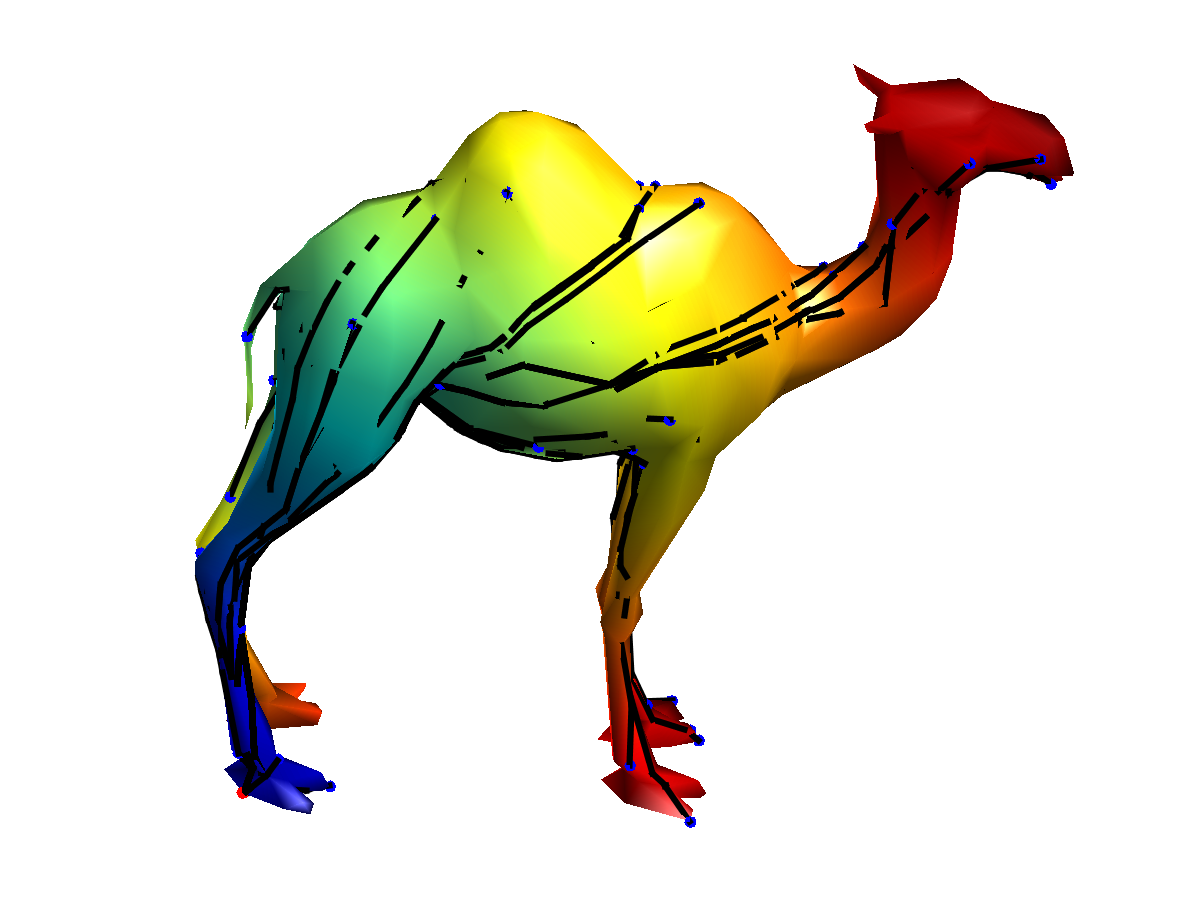The geodesic distance matrix $\de \in \RR^{N \times N}$ is defined as $$\forall i,j=1,\ldots,N, \quad \de_{i,j} = d(x_i,x_j).$$

Exercise 1

Compute the geodesic distance matrix $\de$. It is going to take some of time. progressbar(i,N);

In :
exo1()

In :
%% Insert your code here.


## Bending Invariant with Strain Minimization¶

The goal is to compute a set of points $Y = (y_i)_{i=1}^N$ in $\RR^d$, (here we use $d=2$) stored in a matrix $Y \in \RR^{d \times N}$ such that $$\forall i, j, \quad D^2(Y)_{i,j} \approx \de_{i,j}^2 \qwhereq D^2(Y)_{i,j} = \norm{y_i-y_j}^2.$$

Target dimensionality $d$.

In :
d = 3;


This can be achieved by minimzing a $L^2$ loss $$\umin{Y} \norm{ D^2(Y)-\de^2 }^2 = \sum_{i<j} \abs{ \norm{y_i-y_j}^2 - \de_{i,j}^2 }^2.$$

Strain minimization consider instead the following weighted $L^2$ loss (so-called strain) $$\umin{Y \in \RR^{d \times N} } \text{Strain}(Y) = \norm{ J ( D^2(Y)-\de^2 ) J }^2$$ where $J$ is the so-called centering matrix $$J_{i,j} = \choice{ 1-1/N \qifq i=j, \\ -1/N \qifq i \neq j. }$$

In :
J = eye(N) - ones(N)/N;


Using the properties of squared-distance matrices $D^2(Y)$, one can show that $$\norm{ J ( D^2(Y)-\de^2 ) J }^2 = \norm{ Y Y^* - K }^2 \qwhereq K = - \frac{1}{2} J \de^2 J.$$

In :
K = -1/2 * J*(delta.^2)*J;


The solution to this (non-convex) optimization problem can be computed exactly as the rows of $Y$ being the two leading eigenvectors of $K$ propery rescaled.

In :
opt.disp = 0;
[Y, v] = eigs(K, d, 'LR', opt);
Y = Y .* repmat(sqrt(diag(v))', [N 1]);
Y = Y';


Display the bending invariant surface.

In :
clf;
plot_mesh(Y,F, options);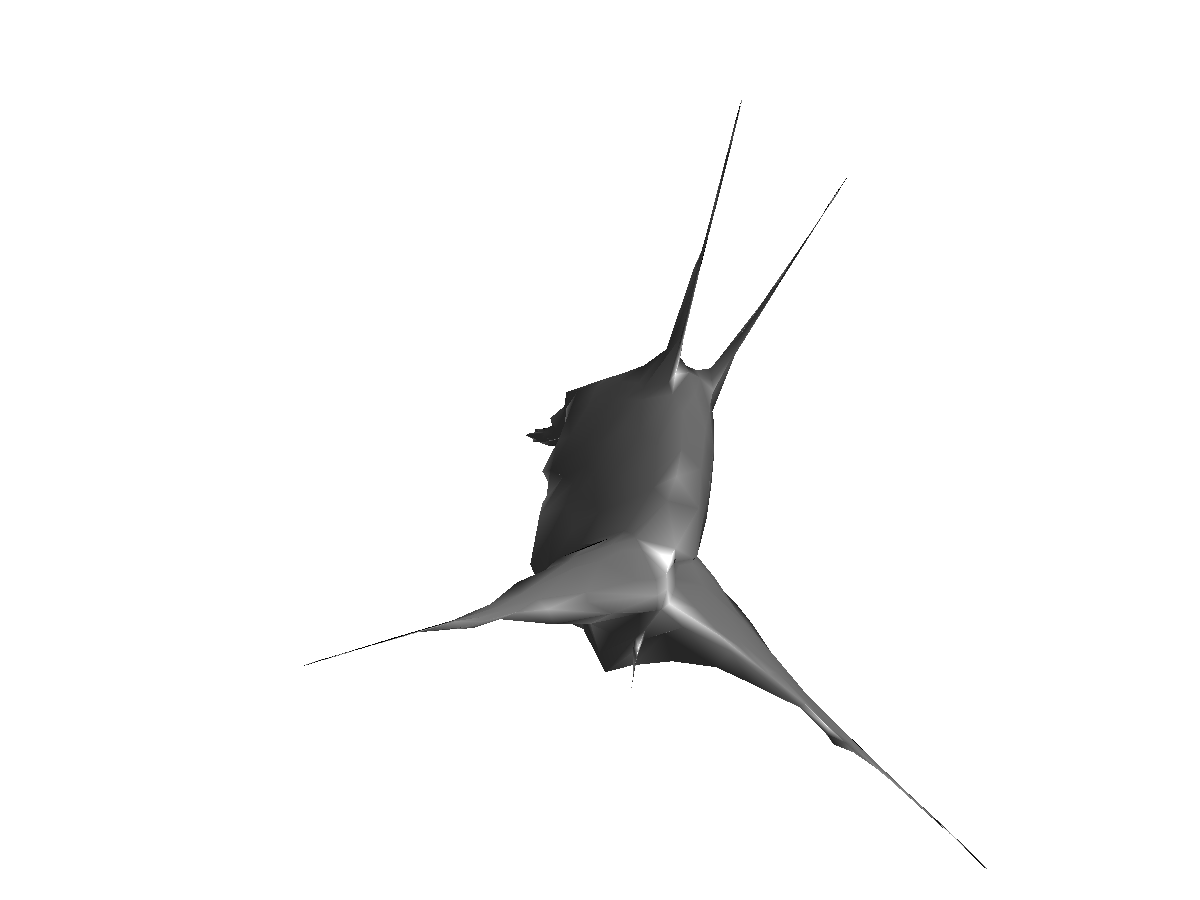## Bending Invariant with Stress Minimization¶

The stress functional does not have geometrical meaning. An alternative MDS method directly minimizes a geometric loss, the so-called Stress $$\umin{Y \in \RR^{d \times N} } \text{Stress}(Y) = \norm{ D(Y)-\de }^2 = \sum_{i<j} \abs{ \norm{y_i-y_j} - \de_{i,j} }^2.$$ It is possible to find a local minimizer of this energy by various descent algorithms, as initially proposed by Kruskal64

In :
Stress = @(d)sqrt( sum( abs(delta(:)-d(:)).^2 ) / N^2 );


Operator to compute the distance matrix $D(Y)$.

In :
D = @(Y)sqrt( repmat(sum(Y.^2),N,1) + repmat(sum(Y.^2),N,1)' - 2*Y'*Y);


The SMACOF (Scaling by majorizing a convex function) algorithm solves at each iterations a quadratic energy, that is guaranteed to diminish the value of the Strain. It was introduced by Leeuw77

It computes iterates $X^{(\ell)}$ as $$X^{(\ell+1)} = \frac{1}{N} X^{(\ell)} B(D(X^{(\ell)}))^*,$$ where $$B(D) = \choice{ -\frac{\de_{i,j}}{D_{i,j}} \qifq i \neq j, \\ -\sum_{k} B(D)_{i,k} \qifq i = j. }$$

Initialize the positions for the algorithm.

In :
Y = V;


Operator $B$.

In :
remove_diag = @(b)b - diag(sum(b));
B = @(D1)remove_diag( -delta./max(D1,1e-10) );


Update the positions.

In :
Y = Y * B(D(Y))' / N;


Exercise 2

Perform the SMACOF iterative algorithm. Save in a variable |s(l)| the values of Stress$( X^{(\ell)} )$.

In :
exo2()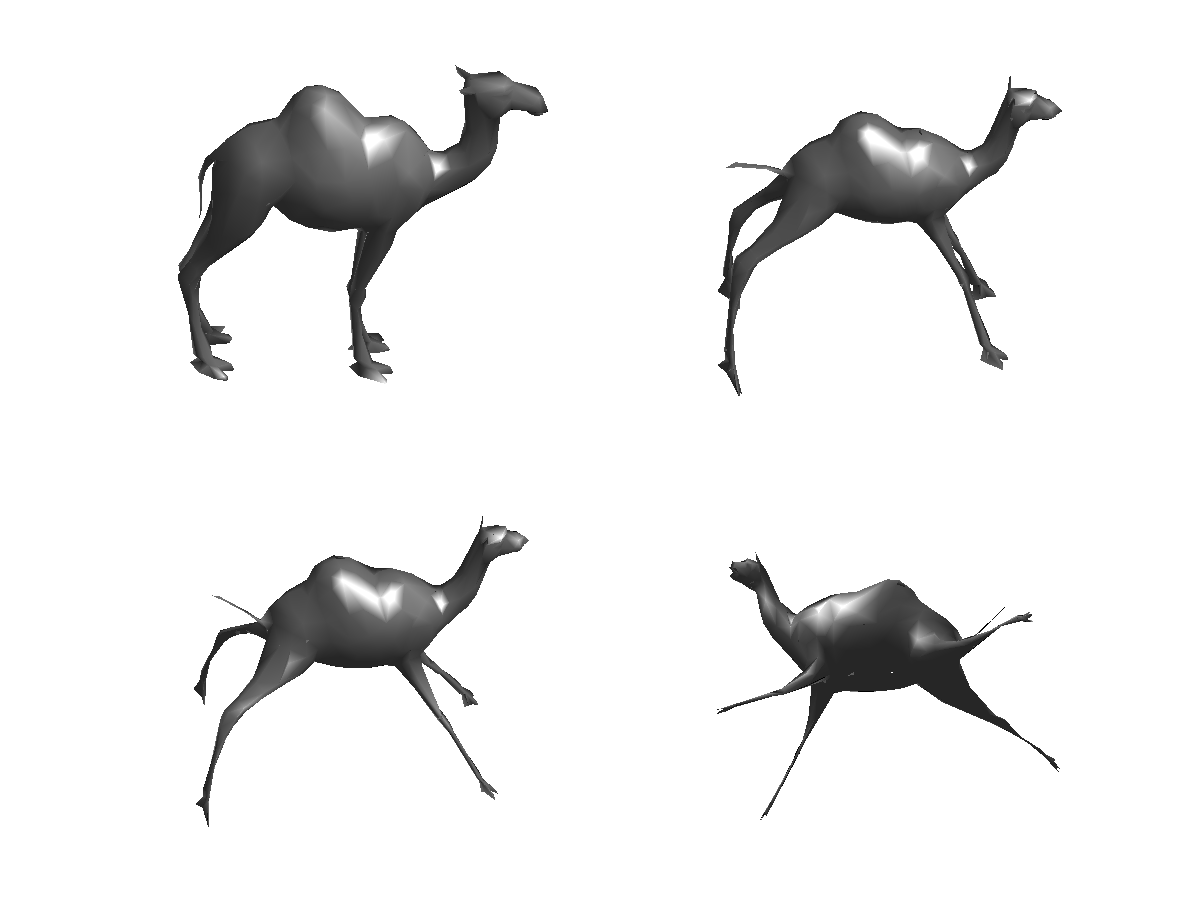In :
%% Insert your code here.


Plot stress evolution during minimization.

In :
clf;
plot(s, '.-', 'LineWidth', 2, 'MarkerSize', 20);
axis('tight');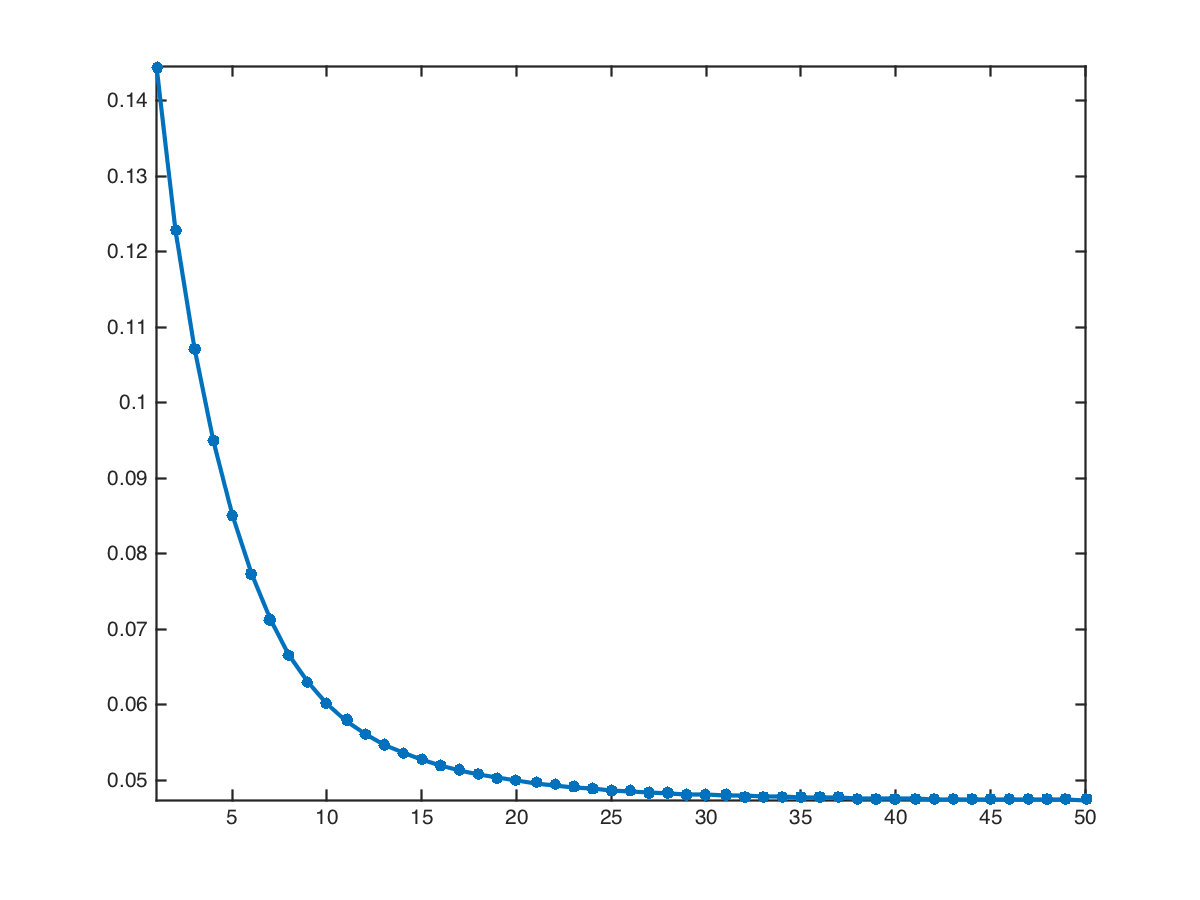Plot the optimized invariant shape.

In :
clf;
plot_mesh(Y,F, options);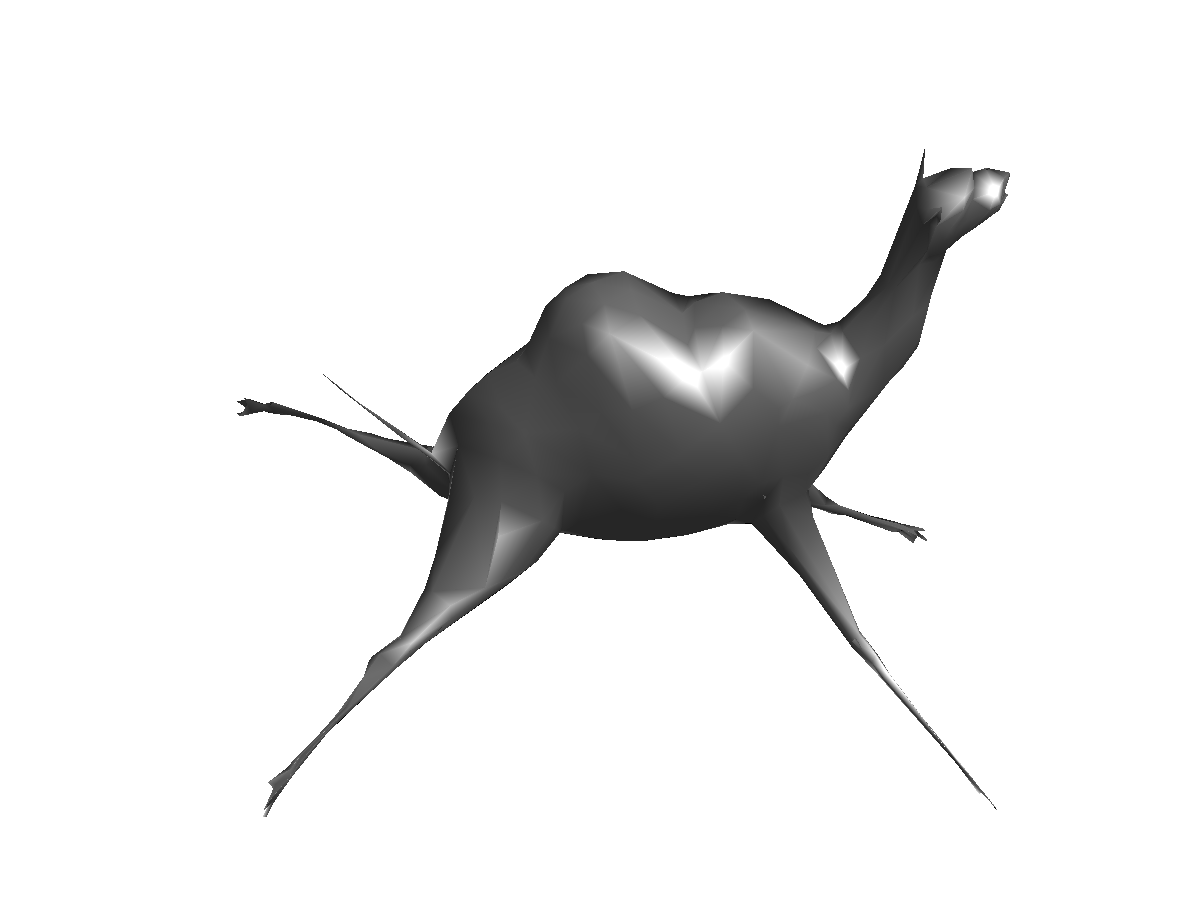## Surface Retrieval with Bending Invariant.¶

One can compute a bending invariant signature for each mesh in a library of 3D surface.

Isometry-invariant retrival is then perform by matching the invariant signature.

Exercise 3

Implement a surface retrival algorithm based on these bending invariants. o correction for this exercise.

In :
exo3()

In :
%% Insert your code here.